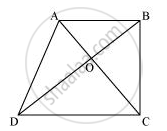# Diagonals AC and BD of a trapezium ABCD with AB || DC intersect each other at O. Prove that ar (AOD) = ar (BOC). - Mathematics

Diagonals AC and BD of a trapezium ABCD with AB || DC intersect each other at O. Prove that ar (AOD) = ar (BOC).

#### SolutionIt can be observed that ΔDAC and ΔDBC lie on the same base DC and between the same parallels AB and CD.

∴ Area (ΔDAC) = Area (ΔDBC)

⇒ Area (ΔDAC) − Area (ΔDOC) = Area (ΔDBC) − Area (ΔDOC)

⇒ Area (ΔAOD) = Area (ΔBOC)

Concept: Corollary: Triangles on the same base and between the same parallels are equal in area.
Is there an error in this question or solution?
Chapter 9: Areas of Parallelograms and Triangles - Exercise 9.3 [Page 163]

#### APPEARS IN

NCERT Class 9 Maths
Chapter 9 Areas of Parallelograms and Triangles
Exercise 9.3 | Q 10 | Page 163

Share